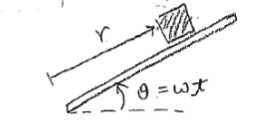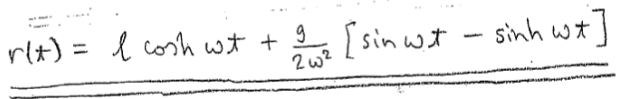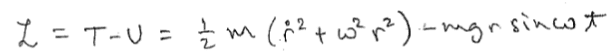# Lagrangian Mechanics with one constraint

## Homework StatementI'm supposed to find the normal force acting on the box by the slab as a function of time. The problem is I don't know what the constraint is. I can't find the relation between r and theta that adds the two up to zero.

## Homework EquationsLagrangian equation.

## The Attempt at a Solution

I tried constraint f(r, theta , t) = theta and got lamda = mr (gcos(theta) + r theta(double dot) ) = mgrcos(theta) since omega is constant, but still couldn't get the right answer.

#### Attachments

TSny
Homework Helper
Gold Member
Hello, and welcome to PF.

If you know ##r## and ##\theta## as functions of time, then I don't understand why you would need to bother with setting up the Lagrangian. Did you state the problem word-for-word as given to you?

Hello, and welcome to PF.

If you know ##r## and ##\theta## as functions of time, then I don't understand why you would need to bother with setting up the Lagrangian. Did you state the problem word-for-word as given to you?
Sorry for the late reply. I was able to figure it out. Thank you.